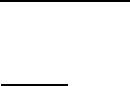Study Guides (380,000)
US (220,000)
Rutgers (3,000)
All (30)

# study guide 2

Department
Mathematics
Course Code
01:640:135
Professor
All

This preview shows half of the first page. to view the full 3 pages of the document.1A
(6) 1. Compute the derivatives of the following functions:
a) xe
cos x
b) tan
3
(x
3
)
(8) 2. Compute the following limits:
a) lim
x!0
e
5x
5x1
x
2
b) lim
x!1
x
2
+1
x+1
(13) 3. Find an equation for the line tangent to the graph of lny+x
3
+2xy = 10 at the point
(2;1).
(8) 4. A certain function f(x) is dened and dierentiable for all real numbers x. If f(1) = 2
and jf
0
(x)j  3 for 1 <x<3, what is the largest possible value of f(3)? What is the